0%

\begin{aligned} v_{*}(s) &=\max _{a} \mathbb{E}\left[R_{t+1}+\gamma v_{*}\left(S_{t+1}\right) \mid S_{t}=s, A_{t}=a\right] \\ &=\max _{a} \sum_{s^{\prime}, r} p\left(s^{\prime}, r \mid s, a\right)\left[r+\gamma v_{*}\left(s^{\prime}\right)\right], \text { or } \end{aligned}

\begin{aligned} q_{*}(s, a) &=\mathbb{E}\left[R_{t+1}+\gamma \max _{a^{\prime}} q_{*}\left(S_{t+1}, a^{\prime}\right) \mid S_{t}=s, A_{t}=a\right] \\ &=\sum_{s^{\prime}, r} p\left(s^{\prime}, r \mid s, a\right)\left[r+\gamma \max _{a^{\prime}} q_{*}\left(s^{\prime}, a^{\prime}\right)\right] \end{aligned}

## 策略评估

\begin{aligned} v_{\pi}(s) & \doteq \mathbb{E}_{\pi}\left[G_{t} \mid S_{t}=s\right] \\ &=\mathbb{E}_{\pi}\left[R_{t+1}+\gamma G_{t+1} \mid S_{t}=s\right] \\ &=\mathbb{E}_{\pi}\left[R_{t+1}+\gamma v_{\pi}\left(S_{t+1}\right) \mid S_{t}=s\right] \\ &=\sum_{a} \pi(a \mid s) \sum_{s^{\prime}, r} p\left(s^{\prime}, r \mid s, a\right)\left[r+\gamma v_{\pi}\left(s^{\prime}\right)\right] \end{aligned}

\begin{aligned} v_{k+1}(s) & \doteq \mathbb{E}_{\pi}\left[R_{t+1}+\gamma v_{k}\left(S_{t+1}\right) \mid S_{t}=s\right] \\ &=\sum_{a} \pi(a \mid s) \sum_{s^{\prime}, r} p\left(s^{\prime}, r \mid s, a\right)\left[r+\gamma v_{k}\left(s^{\prime}\right)\right] \end{aligned}

$$v_{k}=v_{\pi}$$是一个不动点（通过巴拿赫不动点定理来证明），当$$k \rightarrow \infty$$时，$$\{v_k\}$$能够收敛到$$v_\pi$$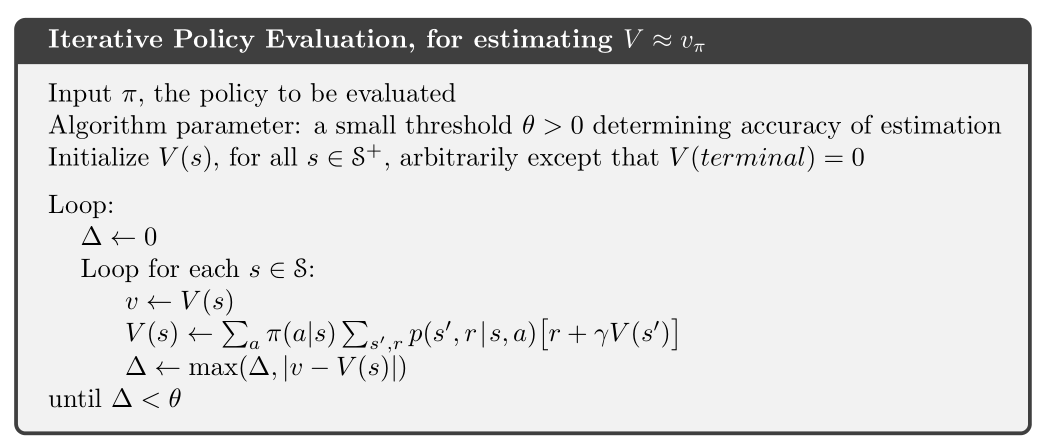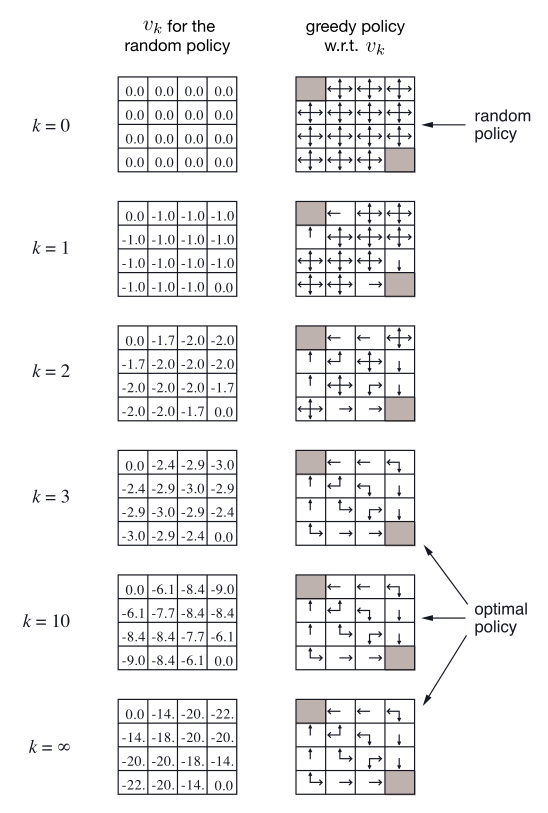## 策略改进

$q_{\pi}\left(s, \pi^{\prime}(s)\right) \geq v_{\pi}(s)$

\begin{aligned} v_{\pi}(s) & \leq q_{\pi}\left(s, \pi^{\prime}(s)\right) \\ &=\mathbb{E}\left[R_{t+1}+\gamma v_{\pi}\left(S_{t+1}\right) \mid S_{t}=s, A_{t}=\pi^{\prime}(s)\right] \\ &=\mathbb{E}_{\pi^{\prime}}\left[R_{t+1}+\gamma v_{\pi}\left(S_{t+1}\right) \mid S_{t}=s\right] \\ & \leq \mathbb{E}_{\pi^{\prime}}\left[R_{t+1}+\gamma q_{\pi}\left(S_{t+1}, \pi^{\prime}\left(S_{t+1}\right)\right) \mid S_{t}=s\right] \\ &=\mathbb{E}_{\pi^{\prime}}\left[R_{t+1}+\gamma \mathbb{E}_{\pi^{\prime}}\left[R_{t+2}+\gamma v_{\pi}\left(S_{t+2}\right) \mid S_{t+1}, A_{t+1}=\pi^{\prime}\left(S_{t+1}\right)\right] \mid S_{t}=s\right] \\ &=\mathbb{E}_{\pi^{\prime}}\left[R_{t+1}+\gamma R_{t+2}+\gamma^{2} v_{\pi}\left(S_{t+2}\right) \mid S_{t}=s\right] \\ & \leq \mathbb{E}_{\pi^{\prime}}\left[R_{t+1}+\gamma R_{t+2}+\gamma^{2} R_{t+3}+\gamma^{3} v_{\pi}\left(S_{t+3}\right) \mid S_{t}=s\right] \\ & \vdots \\ & \leq \mathbb{E}_{\pi^{\prime}}\left[R_{t+1}+\gamma R_{t+2}+\gamma^{2} R_{t+3}+\gamma^{3} R_{t+4}+\cdots \mid S_{t}=s\right] \\ &=v_{\pi^{\prime}}(s) \end{aligned}

\begin{aligned} \pi^{\prime}(s) & \doteq \underset{a}{\arg \max } q_{\pi}(s, a) \\ &=\underset{a}{\arg \max } \mathbb{E}\left[R_{t+1}+\gamma v_{\pi}\left(S_{t+1}\right) \mid S_{t}=s, A_{t}=a\right] \\ &=\underset{a}{\arg \max } \sum_{s^{\prime}, r} p\left(s^{\prime}, r \mid s, a\right)\left[r+\gamma v_{\pi}\left(s^{\prime}\right)\right] \end{aligned}

## 策略迭代

$\pi_{0} \stackrel{\mathrm{E}}{\longrightarrow} v_{\pi_{0}} \stackrel{\mathrm{I}}{\longrightarrow} \pi_{1} \stackrel{\mathrm{E}}{\longrightarrow} v_{\pi_{1}} \stackrel{\mathrm{I}}{\longrightarrow} \pi_{2} \stackrel{\mathrm{E}}{\longrightarrow} \cdots \stackrel{\mathrm{I}}{\longrightarrow} \pi_{*} \stackrel{\mathrm{E}}{\longrightarrow} v_{*}$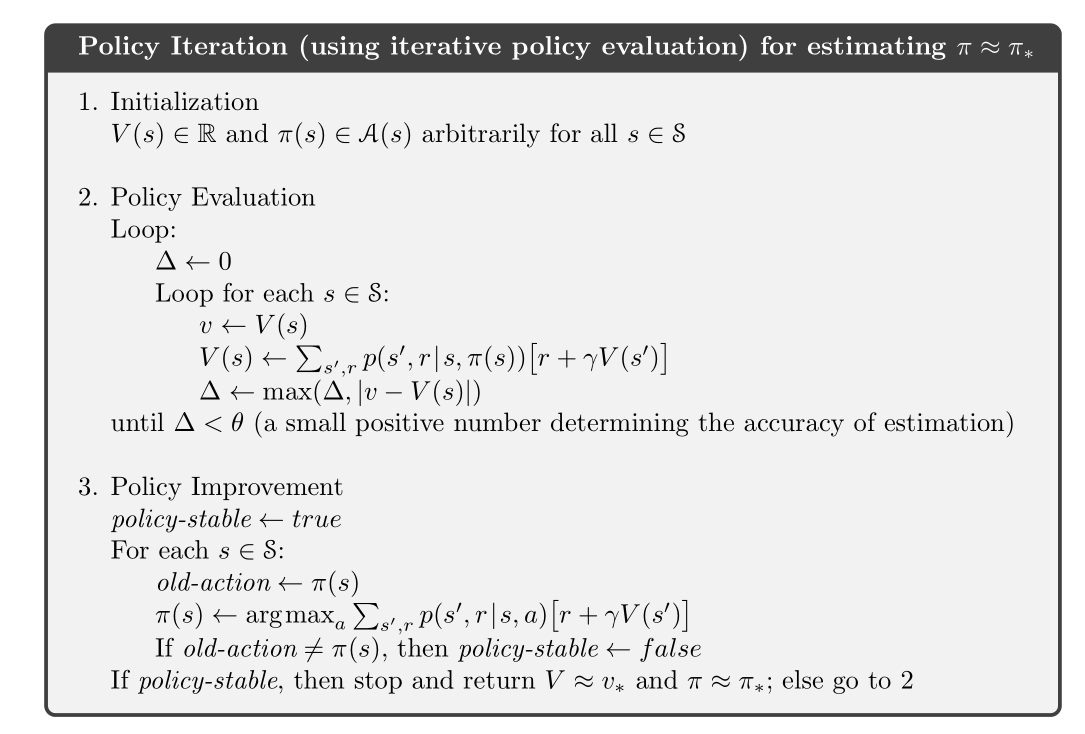## 价值迭代

\begin{aligned} v_{k+1}(s) & \doteq \max _{a} \mathbb{E}\left[R_{t+1}+\gamma v_{k}\left(S_{t+1}\right) \mid S_{t}=s, A_{t}=a\right] \\ &=\max _{a} \sum_{s^{\prime}, r} p\left(s^{\prime}, r \mid s, a\right)\left[r+\gamma v_{k}\left(s^{\prime}\right)\right] \end{aligned}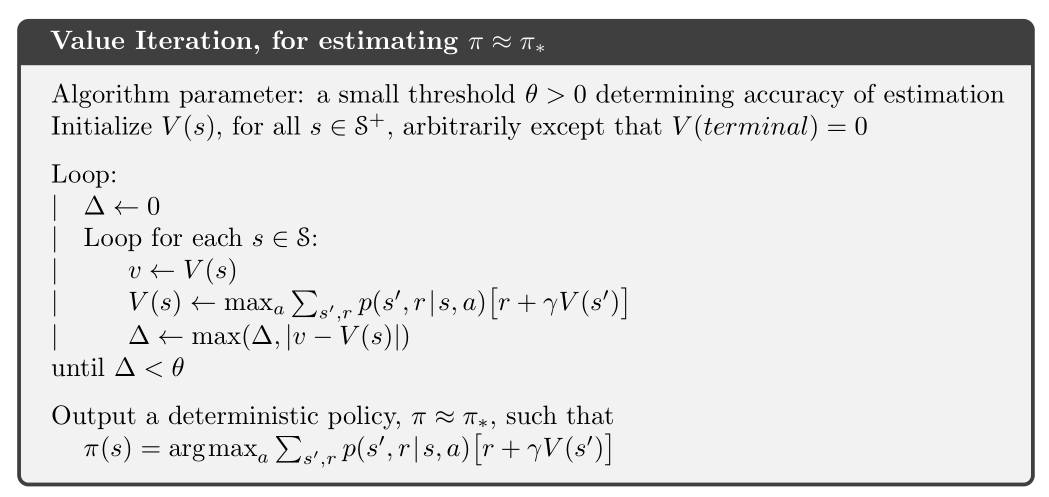## 广义策略迭代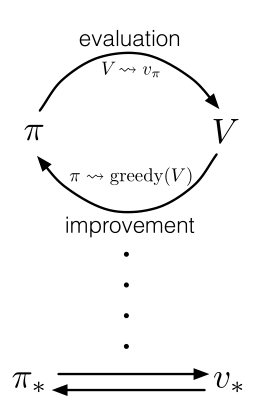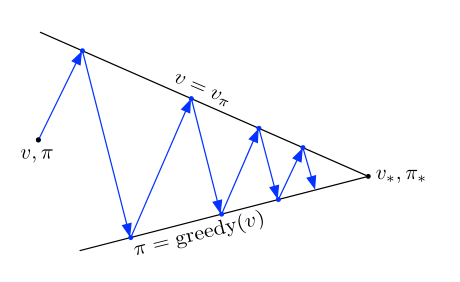## 动态规划的效率

DP算法有时会因为维度灾难而被认为缺乏实用性。集合太大确实会带来一些困难，但是这是问题本身的困难，而非DP作为一种解法所带来的困难。事实上，相比于直接搜索和线性规划，DP更加适合解决大规模状态空间的问题。

If you like my blog, please donate for me.